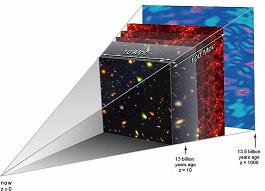What is ‘z’?     z’ is the symbol conventionally used to quantify redshift.

The Universe is Expanding

The Cosmological Redshift is a redshift caused by the expansion of space.

Because of this, once you are looking 'quite a long way away', everything in all directions is moving away from us. Things further away from us are moving faster away from us. On large scales, this is a sensible way to measure the distance.

The Doppler Effect

Stars, galaxies and quasars all emit energy that travels through space in the form of electromagnetic waves – from short x-ray wavelengths, through intermediate wavelengths we see as light, to longer radio wavelengths.

When a light source is moving away from us, the Doppler effect ‘reddens’ the light as we see it – it is shifted to longer wavelengths. One way of thinking of this is to imagine the light waves being 'stretched' as the retreating object pulls away. Red light (at one end of the visible spectrum) has longer wavelengths than blue light (at the other), and so the 'stretching' effect is described as a reddening.

A Distance Scale

We know the wavelength of spectral features when they are emitted, and so when we observe a distant object, we can measure just how much the wavelengths have been changed.

If the observed wavelength is as much as double what we know it must have been when the light was emitted, this extreme redshift tells us that when the light was emitted, the universe was only half the size it is now. That's a long way away, and a long time ago.

z Defined

A quantifiable measure of redshift is the ratio of the wavelength of received light to the wavelength when it was emitted. For example, a distant galaxy may be emitting hydrogen light at the ultra-violet wavelength of 121.5 nanometers, but we may observe this emission at a wavelength of 607.5 nanometers – five times the emitted wavelength.  The ratio is thus 5.

'z' is that number, minus 1. In our example, subtract 1 from this ratio gives us 4 and so this distant galaxy has a redshift of 4.

How Far Can We See?

The most distant objects detected so far are galaxies with redshifts around z = 6. We believe the universe started with the ‘Big Bang’ about 15 billion years ago – we are seeing most of the way back to that.